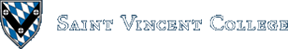Computing & Information Systems    DepartmentSearch CIS Site      Tutorials

## Software Design Using C++

### Introduction

There must be a way to make a decision within a program about whether to execute certain statements or not, or perhaps to decide whether to do one section of code or to do another section instead. We examine how to use the C++ `if` and `switch` constructs to make choices about what to do during the execution of a program.

### An All or Nothing Choice

Here the choice is whether to do a section of code or to skip it entirely. For example, consider the following:

 `````` if (Num > 0) Num = Num * 10; cout << Num << endl; ``````

If the value in `Num` is positive, we assign into it 10 times its value. Then we go on to whatever follows next after the `if` statement. In this case, we print out the value of `Num`. Note that the code that is selected is the single statement following the `if (CONDITION)` construct. Should you want to have multiple statements governed by the `if`, you package up those statements as a block by placing curly braces around them. We will look at an example of that shortly.

If the value of `Num` is not positive, then the assignment statement is skipped. Control passes on to the output statement that follows. Thus if `Num` has value -2, the above example prints -2, but if `Num` has value 3, it prints 30, because the number gets multiplied by 10.

Now consider the following similar example. This time the `if` selects whether or not to do an entire block of code, which is enclosed in curly braces.

 `````` if (Num > 0) { Num = Num * 10; cout << "Multiplying by 10" << endl; } cout << Num << endl; ``````

Can you tell what this section of code does? If `Num` has value -2, this example prints the -2 on the screen. However, if `Num` has value 3, the following would be printed:

 `````` Multiplying by 10 30 ``````

Note that the code that is controlled by the `if` is indented by 3 spaces. The curly braces should line up vertically. That way one can easily see where this important section of code begins and ends.

See the example choice1.cpp. It shows a small but complete program that contains an all or nothing choice.

The general pattern for using an `if` to handle an all or nothing choice is shown below, with an outline of the `if` used to do this and a flow chart showing the flow of control through this `if` construct. The picture indicates that if the CONDITION is true, the ACTION is taken, but if the CONDITION is false, that ACTION is skipped. Either way, control then passes to whatever follows this `if` construct.

 `````` if (CONDITION) ACTION ``````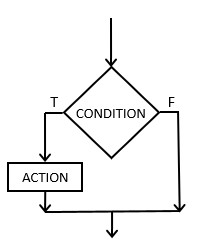### Conditions

True/false conditions are needed to control `if` statements. These can be constructed in a number of ways. One way is by comparing two expressions with a relational operator such as <, >, == (equals), != (not equal), <=, or >=. For example, consider the following:

 `````` x < y Top - Bottom >= 12 Value == Max ``````

The first example condition checks to see if `x` is less than `y`. The second one checks to see if `Top` minus `Bottom` is greater or equal to 12. Finally, the last one checks to see if `Value` and `Max` are equal. Note well that you need two equals signs for an equals comparison. A single equals sign gives an assignment statement, which is quite a different thing!

### To Do This or That

This type of choice is usually made with an `if..else...` construct. If the condition is true, the first section of code is executed. If not, the second section of code (the one following the `else`) is executed. In either case, after the appropriate section of code has been executed, control passes to whatever follows the entire `if..else...` construct. If a section of code for one of the choices consists of more than one statement, then those statements must be packaged up as a block by using curly braces.

The following example shows such a two-way choice. Depending on the value of the variable, either `positive` or `not positive` is printed. Then, either way, a final message is printed.

 `````` if (Num > 0) cout << "positive" << endl; else cout << "not positive" << endl; cout << "In all cases we reach here and print this message."; ``````

See the example choice2.cpp. It shows a complete program that contains this type of choice.

The pattern for using an `if/else` to handle a 2-way choice (a choice between ACTION 1 and ACTION 2) is shown below, with an outline of the `if` used to do this and a flow chart showing the flow of control through this `if` construct. The picture indicates that if the CONDITION is true, ACTION 1 is taken, but if the CONDITION is false, ACTION 2 is taken. Control then passes to whatever follows this `if/else` construct.

 `````` if (CONDITION) ACTION 1 else ACTION 2 ``````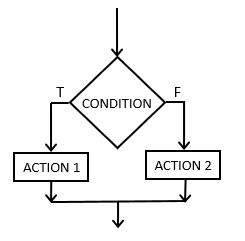### Compound Conditions

Two or more conditions can be combined by using the boolean operators && (AND), || (OR), ! (NOT). NOT reverses the truth value of the condition to which it is applied. The result of ANDing or ORing two conditions is most easily summarized in a truth table as shown below. A AND B is only true when both A and B are true. A OR B is only false when both are false.

A B A AND B A OR B
True True True True
True False False True
False True False True
False False False False

For example, suppose we want to test if a number grade is within the valid range of 0 to 100. This might be done with a compound condition as follows:

 `````` if ((Num >= 0) && (Num <= 100)) { // Num is valid, so we do whatever processing we want to on Num here. // Code for this is not shown. } else cout << "Invalid number grade" << endl; ``````

The above program fragment is equivalent to the following. Note the use of ! for NOT and the || for OR. It uses these to write a condition that checks to see that the value of the variable is not invalid. Note that because of the parentheses the NOT applies to the OR of the two conditions, that is, the NOT applies to `((Num < 0) || (Num > 100))`.

 `````` if (! ((Num < 0) || (Num > 100))) { // Num is valid, so we do whatever processing we want to on Num here. // Code for this is not shown. } else cout << "Invalid number grade" << endl; ``````

The program area4.cpp is a good example of this type of thing. It checks that the length and width of the rectangle are positive numbers before trying to multiply them in order to get the area. This obviously requires two conditions: one for the length and one for the width.

### Boolean Variables

Boolean variables are of type `bool`. Such a variable can at any point take on just one of two possible values: `true` or `false`. Here is an outline of a simple example:

 `````` bool Error; Error = false; if (such and such goes wrong) Error = true; else Do some processing of data; Do some other stuff in all cases; if (Error) cout << "An error has been reported" << endl; else cout << "All is OK" << endl; ``````

In this example, the `Error` variable is used to remember whether or not an error happened. Later, when some output about the status of the program is desired, this variable can be testing in an `if` statement to decide what to print. Boolean variables are great for storing true/false, yes/no types of information. If you want to check if a boolean variable, such as `Error` is not true, use something like this:

 `````` if (! Error) cout << "No error has been found" << endl; else cout << "An error has been reported" << endl; ``````

### Multiway Choices

Sometimes you need to decide among multiple possibilities. See the program choice3.cpp for an example where we decide which of three sections of code should be executed. This requires at least two conditions. The `extended if` itself looks like this:

 `````` if (Num > 0) cout << " is positive" << endl << endl; else if (Num == 0) cout << " is zero" << endl << endl; else cout << " is negative" << endl << endl; ``````

Note that the `else if` combination is new. How does this example work? If the first condition is true, the first section of code is executed and the rest is skipped. If not, the second condition is tried. If it is true, the second section of code is executed and the rest is skipped. If the second condition is found to be false, the third section of code is executed. No matter which of the 3 sections of code is executed, control then passes to whatever follows the `extended if` construct. Note the indenting pattern. The indenting of the 3 sections of code makes it easy to see at a glance what the 3 choices are.

The general pattern for using an `extended if` to handle a 3-way choice (a choice between ACTION 1, ACTION 2, and ACTION 3) is shown below, with an outline of the `extended if` used to do this and a flow chart showing the flow of control through this construct. The picture indicates that if CONDITION A is true, ACTION 1 is taken, but if CONDITION A is false, CONDITION B is then examined. At that point, if CONDITION B is true, ACTION 2 is taken, but if CONDITION B is false (and remember that CONDITION A was false for us to even reach this point in the code), then ACTION 3 is taken. No matter which action is executed, control then passes to whatever follows this `extended if` construct.

 `````` if (CONDITION A) ACTION 1 else if (CONDITION B) ACTION 2 else ACTION 3 ``````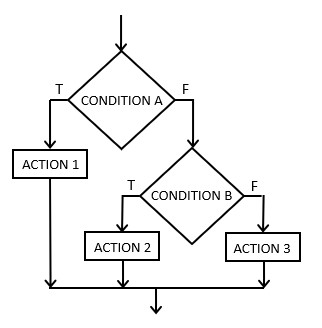The `extended if` can be continued further to handle as many choices as you like. For example, suppose you ask the user to press a letter to indicate a selection from a program menu. You can then use an `extended if` to select the correct section of code to execute. This might look like the following:

 `````` char Choice; cout << "Enter the letter for your choice: "; cin >> Choice; if (Choice == 'A') DoA(); else if (Choice == 'B') DoB(); else if (Choice == 'C') DoC(); else if (Choice == 'D') DoD(); ``````

The above code assumes that we have written functions called `DoA`, `DoB`, etc. to carry out the desired actions. We could add an `else` clause to catch anything other than A, B, C, or D and write out an error message in such a case, if we wanted. You might also wonder why we didn't write the above code using separate `if` statements. This could, in fact, be done and would look like the following:

 `````` char Choice; cout << "Enter the letter for your choice: "; cin >> Choice; if (Choice == 'A') DoA(); if (Choice == 'B') DoB(); if (Choice == 'C') DoC(); if (Choice == 'D') DoD(); ``````

The blank lines between the separate `if` statements are not necessary, but they help to make it clear that these are indeed separate `if` statements. Which of these two ways of handling the choice of A, B, C, or D is better? Both methods clearly work. One way to tell is to draw a decision tree for each method, showing in each case the choices that are possible: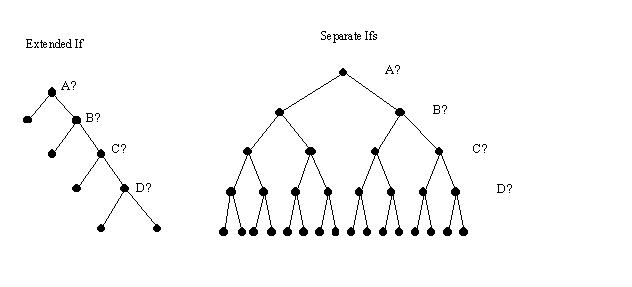In each tree, the conditions are labelled with a question mark. If the answer to a question is yes, then the branch to the left is taken. If the answer to a question is no, then the branch to the right is taken. In the decision tree for the `extended if` it is clear that in some cases only one condition is checked, in other cases two conditions are checked before the correct code is selected, in others three conditions are checked, and sometimes all four conditions are checked. On average, though, fewer than all 4 conditions are tested. In the other method, all 4 conditions are always checked. This is needless and a waste of time. Once we know that the variable has A in it, there is no need to check to see if it is a B, etc. Thus our decision trees have helped us to conclude that the `extended if` pattern is more efficient that `separate ifs`. In general, you should not use `separate ifs` when an `extended if` can be used instead.

The program grade1.cpp is an example that uses an `extended if` pattern in order to convert a numeric grade to the correct letter grade. It also uses one compound condition.

### The Switch

This construct provides an alternate way to handle multiway choices when the conditions are based on equality. For example, in the following code we want to choose to call one of 4 functions based on whether variable `Choice` equals A, B, C, or D (or their lower case equivalents).

 `````` char Choice; cout << "Enter the letter for your choice: "; cin >> Choice; switch(Choice) { case 'A': case 'a': DoOptionA(); break; case 'B': case 'b': DoOptionB(); break; case 'C': case 'c': DoOptionC(); break; case 'D': case 'd': DoOptionD(); } ``````

Note that everything in the switch is enclosed in curly braces, but that braces are not needed around the code blocks among which we are choosing. When the switch is executed it checks the value of the variable listed in the `switch()` with each case in order. If a match is found, it then executes the code below that case. Typically the section of code ends with a `break` statement which takes us to whatever follows the entire switch construct. If there is no `break` at the end of the section of code, control passes right into the next section of code, whether or not the variable matches the case at the head of this section of code! That is why the `break` statements above are so important. There is no need of a break after the code for the last case as we will exit from the switch construct after executing this code anyway. If the value of the variable doesn't match any of the cases in the switch construct, then the entire construct is skipped over.

Let's look at a variation on the above example in which we use an integer variable instead of a character variable. Although we could read in 1, 2, 3, or 4 into a character variable, it would be more typical to read them into an integer variable. (Besides, we could not read in a multi-character item like 12 into a single-character variable, so an integer variable is better here.) Note that literal character values are written with single quotes around them as in '2', whereas literal integer or float values are written without quotes as in 2.

 `````` int Num; cout << "Enter the number for your choice: "; cin >> Num; switch(Num) { case 1: DoOption1(); break; case 2: DoOption2(); break; case 3: DoOption3(); break; case 4: DoOption4(); break; default: cout << "Invalid number was entered" << endl; } ``````

This example also illustrates the `default` case. If the value of `Num` doesn't match any of the other cases, then the code for the `default` case is executed.

Back to the main page for Software Design Using C++

Author: Br. David Carlson with contributions by Br. Isidore Minerd
Last updated: September 01, 2020
Disclaimer